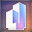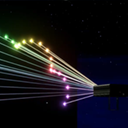Tower Unite

### Tower Unite128 ratingsOfficial Guide: Laser Expression Editor Walk-through
By MacDGuy and 1 collaborators
This guide will cover the basics of the Laser Projector expression editor and how to use its various functions! It's an exciting guide filled with drama, betrayal, brotherhood, power, and other names for sadness.Award
Brief Overview of the Laser Expression Editor
The laser expression editor allows you to write math expressions to control the projection pattern on the laser projector.
Expression Reference
Outputs
The outputs are the parameters that control the look and behaviour of each individual beam. This includes where they point at, as well as their colours. Be sure to define each of the following outputs in your expressions.
Output
Description
x'
The output horizontal coordinate of the laser beam, ranging from -100 (left) to 100 (right).
y'
The output vertical coordinate of the laser beam, ranging from -100 (down) to 100 (up).
h
The beam hue, ranging from to 360°. This value is wrapped, so the value is always remapped to this range. 0 means red, 120 means green, and 240 means blue. All values inbetween are interpolated, so you can pick any colour in the spectrum.
s
The beam saturation, ranging from 0 to 1. 0 results in a white beam, and 1 results in a fully saturated colour.
v
The beam value (brightness), ranging from 0 to 1. 0 results in the beam being invisible, and 1 results in a fully visible beam.

Inputs
These are parameters that you can feed into the output expressions. Some of the parameters give an indication of which laser beam it is, while others can be used to cause the beams to move dynamically.
Input
Description
x
The input horizontal coordinate taken from the projection base (shape or grid), ranging from -100 (left) to 100 (right).
y
The input vertical coordinate taken from the projection base (shape or grid), ranging from -100 (down) to 100 (up).
index
The unique number associated with the current beam. The index on the first beam is 0, and the last beam equal to the number of beams minus 1.
count
The total amount of laser beams in the current projection. In most shapes this is equal to 32.
fraction
This is equal to the index of the beam divided by the total amount of beams.
pi
3.14159265358979323846264338327950288419716...
tau
Two times pi. Useful for quickly converting radians per second to Hertz, when using functions such as sin(), cos(), and tan().
time
The current time, in seconds, on the local system (your computer).
projectionTime
The time, in seconds, since the expression was activated.
projectionStartTime
The local time, in seconds, when the expression was activated.

Functions
Function
Description
sin(<angle>)
Returns the sine of 'angle'.
cos(<angle>)
Returns the cosine of 'angle'.
tan(<angle>)
Returns the tangent of 'angle'.
asin(<float>)
Returns the arcsine of 'float'.
acos(<float>)
Returns the arccosine of 'float'.
atan(<float>)
Returns the arctangent of 'float'.
atan2(<x>, <y>)
Returns the arctangent of 'y'/'x' with consideration for divide-by-zero edge cases.
sqrt(<float>)
Returns the square-root of 'float'.
min(<a>,<b>)
Returns the smaller value of 'a' or 'b'.
max(<a>,<b>)
Returns the larger value of 'a' or 'b'
floor(<float>)
Returns the value 'float' rounded down to the nearest whole number less than 'float'
ceil(<float>)
Returns the value 'float' rounded up to the nearest whole number greater than 'float'
round(<float>)
Returns the value 'float' rounded to the nearest whole number.
abs(<float>)
Returns the absolute value of 'float' ('float' < 0 ? -'float' : 'float').
rand()
Returns a random number between 0.0 and 1.0.
if(<float>, <a>, <b>)
Returns 'a' if 'float' is 1 or greater otherwise returns b.
lerp(<frac>, <a>, <b>)
Interpolates between 'a' and 'b' using 'frac' (a*frac + b*(1-frac)).

Operators
Maths and logic. What more is there to say?
Operator
Description
=
Equal. Assigns the value of the right operand to the left operand. You can use this to declare your own variables.
+
Add. Returns the sum of the left and right operands.
-
Subtract. Returns the left minus the right operand.
*
Multiply. Returns the product of the left and right operands.
/
Divide. Returns the left divided by the right operand.
^
Exponent. Returns the left operand to the power of the right operand.
%
Modulo. Returns the remainder of the left operand divided by the right operand.
<
Less than. Returns 1 when the left operand is less than the right, otherwise returns 0.
>
Greater than. Returns 1 when the left operand is greater than the right, otherwise returns 0.
<=
Less than or equal. Returns 1 when the left operand is less than the right, otherwise returns 0.
>=
Greater than or equal. Returns 1 when the left operand is greater than the right, otherwise returns 0.
==
Equal to. Returns 1 when the left operand is equal to the right, otherwise returns 0.
&
And. Returns 1 if both the left side and right side are true.
|
Or. Returns 1 if either the left side and right side are true.
!
Not. Returns 1 if the expression is false and 0 if the expression is true (inverts the value).
#
Comment. Allows you to put plain text behind it, without getting compiled.
Example Expressions
Simple sine wave
A sine wave is added to the vertical output component of the projector.
#wave parameters: phase = tau*time; #wave movement (1 cycle per second) frequency = 0.05; #wave width amp = 10; #wave height #output: x' = x; y' = y + amp * sin( frequency*x + phase );

Scales the shape up and down instantaneously.
#cycle duration in seconds rate = .2; #wrap time to cycle duration (ramainder of time / rate) wraptime = time % rate; #'>' operator gives 1 if wraptime > rate/2, otherwise 0 isscaleddown = wraptime > rate/2; #do scaling scaledownamount = .5; scale = 1 - isscaleddown * scaledownamount; #output: x' = x * scale; y' = y * scale;

Color wheel
Changes the color of each laser based on its angle from center.
#atan2 returns the angle between 0,0 and y,x #atan2's range is (-pi,pi], here we convert to (0, 1] #we first divide by 'pi' to give us the range (-1, 1] #then we add 1 to get (0, 2] #finally, we divide by 2 to get (0, 1] diskposition = ( 1+atan2(y,x)/pi ) / 2; #scale diskposition by 360 for hue output: colordisk = diskposition * 360; #add time to get the disk rotating #200time is a shortcut for 200 * time: huerot = colordisk + 200time; #output: x' = x; y' = y; h = huerot; s = 1;
3D Rotation
Rotates (x, y, z) by angles (rx, ry, rz)
You can use z' to simulate depth in any way you like.
#projector doesn't have a z value, make one up or set to 0 z = 0; #rotation around each axis: rx = time; ry = time/2; rz = time/4; #cosine and sine values for each rotation axis: cx = cos(rx); sx = sin(rx); cy = cos(ry); sy = sin(ry); cz = cos(rz); sz = sin(rz); #result vector: x' = x*(cz*cy) + y*(cy*-sz) + z*sy; y' = x*(-sx*-sy*cz+sz*cx) + y*(-sx*-sy*-sz+cx*cz) + z*-sx*cy; z' = x*(cz*cx*-sy+sz*sx) + y*(-sz*cx*-sy+cz*sx) + z*cx*cy;

Waving three-colour flag
Be a nationalist.

Analog Clock
A clock!
# Construct 3 linearly increasing waves, one for each hand extent = (fraction % (1/3)) * 100; # Convert the seconds to radians on a clock angle = -floor(time) * pi * 2 ; # Calculate the angle of each hand secondAng = angle / 60 + pi/2; minuteAng = angle / (60^2) + pi/2; hourAng = angle / (60^2 * 12) + pi/2; # Plot out the lines for each clock hand secondX = cos(secondAng) * extent; secondY = sin(secondAng) * extent; minuteX = cos(minuteAng) * extent * .8; minuteY = sin(minuteAng) * extent * .8; hourX = cos(hourAng) * extent * 0.5; hourY = sin(hourAng) * extent * 0.5; # Choose the colors for each of the hands secondH = extent; minuteH = 100 + extent; hourH = 200 + extent; # Check if the current beam should be a part of the second, minute, or hour hand isSecond = fraction < (1/3); isMinute = if(isSecond, 0, fraction < (2/3)); # Select the position based on the criteria above x' = if(isSecond, secondX, if(isMinute, minuteX, hourX)); y' = if(isSecond, secondY, if(isMinute, minuteY, hourY)); # And the same for the color h = if(isSecond, secondH, if(isMinute, minuteH, hourH)); s = 1; v = 1;

size = 500; # Plot the tornado points in 3d sidx = index * 0.15; spin = sidx + (time / (sidx ^ 1.2)) * 50; heightRand = (sidx ^ 0.6) * 10 -sin(sidx * 10000) * 8; xp = cos(spin) * sidx; yp = heightRand; zp = sin(spin) * sidx + 500; xp = xp + sin(sidx/10 + time) * 10; zp = zp + cos(sidx/10 + time) * 10; x' = xp; y' = yp + 100; z = zp; x' = x' * size / z; y' = y' * size / z - 145; h = -time*100 + fraction*350; v = 2.5 - z/300; s = v;
lightwo Aug 4, 2022 @ 10:06am
I modified the sine and now it's a big speen speeeeeen

# Suggested: Double Quarter Circle
#wave parameters:
phase = tau*time; #wave movement (1 cycle per second)
frequency = 0.4; #wave width
amp = 10; #wave height

#output:
#x' = x;
x' = x + amp * sin( frequency/2*x + phase+ );
y' = y + amp * sin( frequency*x + phase );
h = projectionTime*100;
s = 1;
v = 1;
Chrnan6710 Apr 3, 2021 @ 2:26pm
Welp, this is my life now
eriolu Jan 20, 2021 @ 4:54am
Time to learn about Sin/Cos signals again x)
john_johnson5 Feb 4, 2020 @ 12:23pm
Time to go back to geometry
PC007 Sep 7, 2019 @ 8:15pm
ThanksRohlik Apr 6, 2019 @ 1:05pm
where do i put these codes?
itsmeowdev Jun 16, 2018 @ 4:23pm
Can you add this color changing beam code?

rate = 10000;

wraptime = time % rate;

x' = x
y' = y
h = wraptime * 170;
s = 1;
v = 1000;
KrystalDisc Feb 1, 2018 @ 6:49pm
Where do I put the code?
Pigeon Dec 14, 2017 @ 8:56am
How can I make the loss meme in this becouse I don't understand a single thing.OwnedMyBe Nov 19, 2017 @ 3:47am
how can i write R A K U N with lazer, can someone teach me